兰州实创装饰非非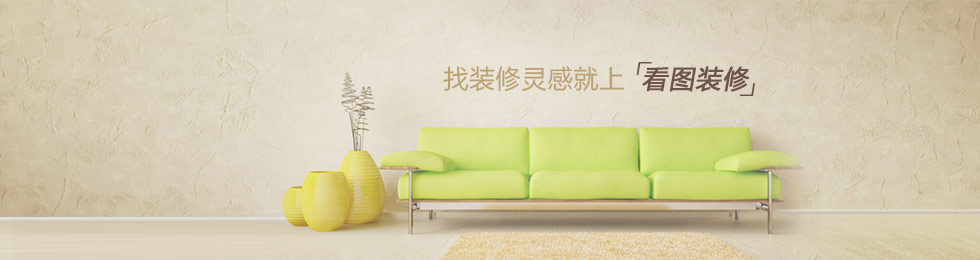兰州实创装饰非非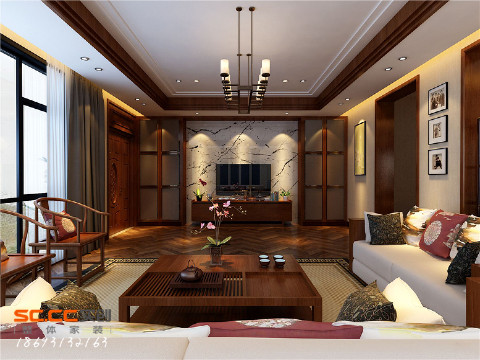装修效果图林隐天下中式风格
• 装修设计
• 装修施工
• 装修完成
• 地区：甘肃  兰州
• 楼盘：林隐天下
• 户型：别墅
• 风格：中式
• 面积：
• 报价：万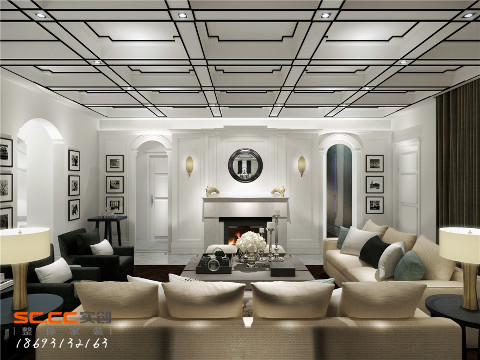兰州薇乐花园新中式风格效果图
• 装修设计
• 装修施工
• 装修完成
• 地区：甘肃  兰州
• 楼盘：薇乐花园
• 户型：别墅
• 风格：中式
• 面积：0.00
• 报价：0.00万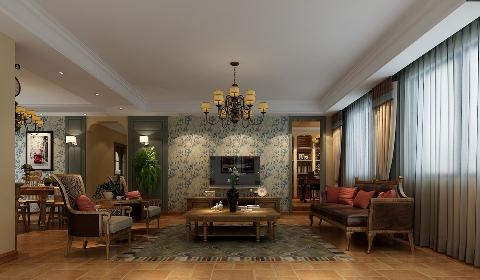兰州碧桂园地中海复古大宅
• 装修设计
• 装修施工
• 装修完成
• 地区：甘肃  兰州
• 楼盘：碧桂园
• 户型：三居
• 风格：现代
• 面积：
• 报价：万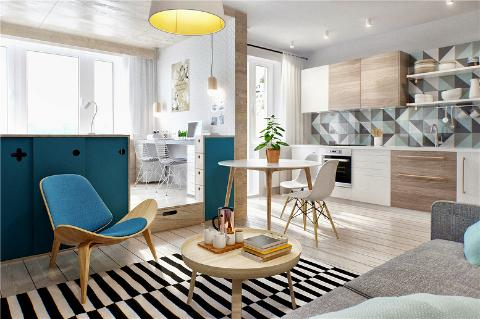豪布斯卡现代简约三居装修
• 装修设计
• 装修施工
• 装修完成
• 地区：甘肃  兰州
• 楼盘：豪布斯卡
• 户型：二居
• 风格：现代
• 面积：
• 报价：万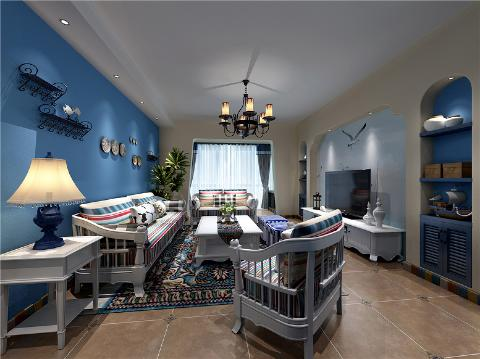兰州万佳润泽园地中海风格
• 装修设计
• 装修施工
• 装修完成
• 地区：甘肃  兰州
• 楼盘：万佳润泽园
• 户型：三居
• 风格：地中海
• 面积：
• 报价：万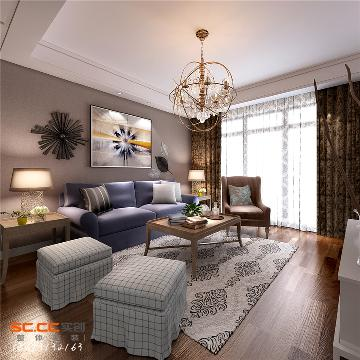兰州金汇广场两居现代时尚
• 装修设计
• 装修施工
• 装修完成
• 地区：甘肃  兰州
• 楼盘：金汇广场
• 户型：二居
• 风格：现代
• 面积：
• 报价：万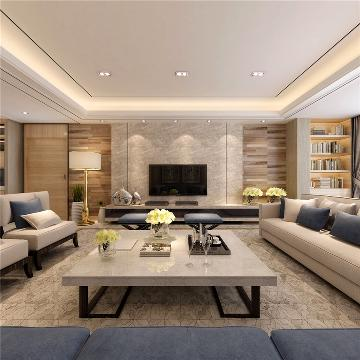和泰馨和园装修效果图简约四居
• 装修设计
• 装修施工
• 装修完成
• 地区：甘肃  兰州
• 楼盘：和泰馨和园
• 户型：四居
• 风格：现代
• 面积：
• 报价：万林隐天下别墅装修欧式梦幻古典
• 装修设计
• 装修施工
• 装修完成
• 地区：甘肃  兰州
• 楼盘：林隐天下
• 户型：别墅
• 风格：欧式
• 面积：
• 报价：万兰州雁乐家苑装修效果图地中海
• 装修设计
• 装修施工
• 装修完成
• 地区：甘肃  兰州
• 楼盘：雁乐家苑
• 户型：三居
• 风格：地中海
• 面积：
• 报价：万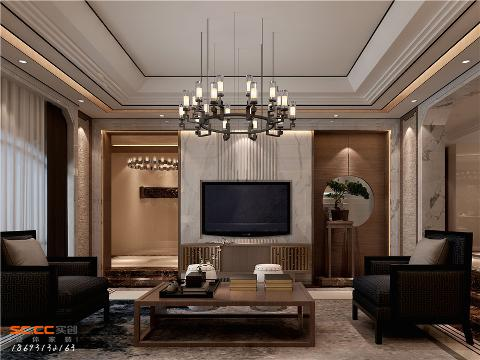保利领秀山别墅装修效果图
• 装修设计
• 装修施工
• 装修完成
• 地区：甘肃  兰州
• 楼盘：保利领秀山
• 户型：三居
• 风格：现代
• 面积：0.00
• 报价：0.00万兰州易大天地欧式风格装修效果图
• 装修设计
• 装修施工
• 装修完成
• 地区：甘肃  兰州
• 楼盘：易大天地
• 户型：三居
• 风格：现代
• 面积：
• 报价：万兰州实创装饰金汇广场装修
• 装修设计
• 装修施工
• 装修完成
• 地区：甘肃  兰州
• 楼盘：金汇广场
• 户型：二居
• 风格：现代
• 面积：
• 报价：万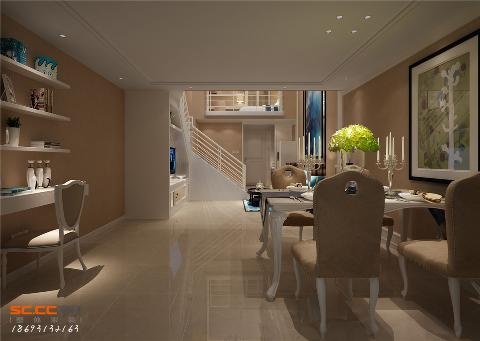兰州碧桂园装修效果图欧式风格
• 装修设计
• 装修施工
• 装修完成
• 地区：甘肃  兰州
• 楼盘：碧桂园
• 户型：三居
• 风格：欧式
• 面积：
• 报价：万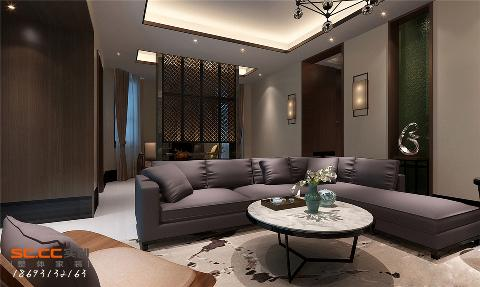兰州中海河山郡新中式效果图
• 装修设计
• 装修施工
• 装修完成
• 地区：甘肃  兰州
• 楼盘：中海河山郡
• 户型：四居
• 风格：中式
• 面积：
• 报价：万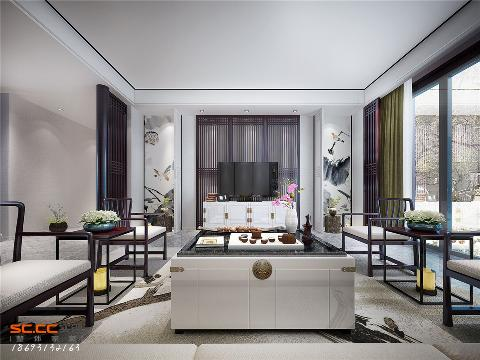兰州林隐天下新中式别墅效果图
• 装修设计
• 装修施工
• 装修完成
• 地区：甘肃  兰州
• 楼盘：林隐天下
• 户型：别墅
• 风格：中式
• 面积：
• 报价：万林隐天下独栋别墅装修效果图
• 装修设计
• 装修施工
• 装修完成
• 地区：甘肃  兰州
• 楼盘：林隐天下
• 户型：别墅
• 风格：现代
• 面积：
• 报价：万兰州实创地中海装修 梦幻蔚蓝
• 装修设计
• 装修施工
• 装修完成
• 地区：甘肃  兰州
• 楼盘：碧桂园
• 户型：三居
• 风格：地中海
• 面积：0.00
• 报价：0.00万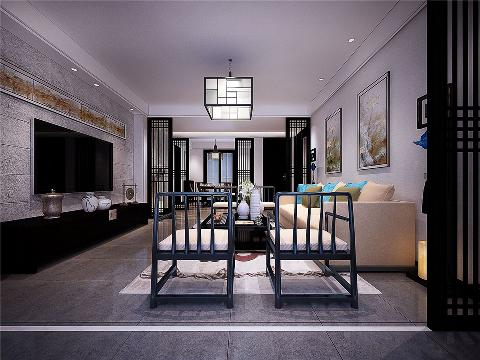兰州碧桂园中式风格大平层
• 装修设计
• 装修施工
• 装修完成
• 地区：甘肃  兰州
• 楼盘：碧桂园
• 户型：四居
• 风格：中式
• 面积：0.00
• 报价：0.00万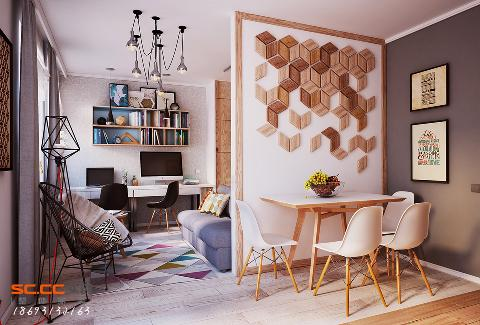兰州碧桂园效果图简约宜家风
• 装修设计
• 装修施工
• 装修完成
• 地区：甘肃  兰州
• 楼盘：碧桂园
• 户型：三居
• 风格：北欧
• 面积：
• 报价：万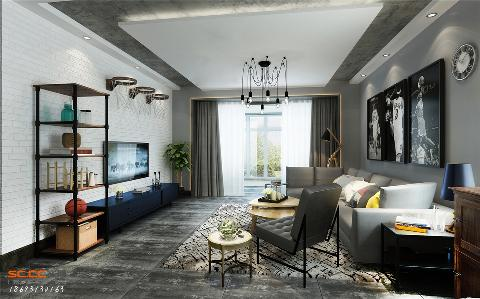兰州新区绿地别墅工业风效果图
• 装修设计
• 装修施工
• 装修完成
• 地区：甘肃  兰州
• 楼盘：绿地
• 户型：别墅
• 风格：现代
• 面积：
• 报价：万

123456 共 15 页